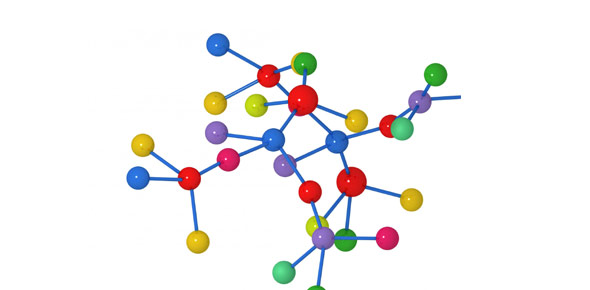Bch211 - Exam 2

46 Questions | Total Attempts: 54SettingsStudy for final

• 1.
True or false:  Enzymes, being proteins, are sensitive to temperature and will be denatured by high temperatures.
• A.

True

• B.

False

• 2.
True or false:  Energy is generally consumed during anabolism
• A.

True

• B.

False

• 3.
True or false:  Aspirin acts as an reversible inhibitor of the COX 2 enzyme
• A.

True

• B.

False

• 4.
Which of the following is a common function of many vitamins in the body?
• A.

Substrates

• B.

Activators

• C.

Apoenzymes

• D.

Coenzymes

• 5.
A metal ion required for enzyme activity is called
• A.

Proenzyme

• B.

A zymogen

• C.

A cofactor

• D.

A substrate

• 6.
Sugars which contain an aldehyde group that can be oxidized are called
• A.

Glycosides

• B.

Simple sugars

• C.

Reducing sugars

• D.

Oxidizing sugars

• 7.
The cyclic form of glucose results from the formation of
• A.

A hemiacetal

• B.

An acetal

• C.

An amide

• D.

An ester

• 8.
The carbohydrate below may be classified as
• A.

A ketohexose

• B.

An aldopentose

• C.

A ketopentose

• D.

An aldohexose

• 9.
The conditions required for maximum enzyme function are called:
• A.

Optimum conditions

• B.

Low pressure, high temperature

• C.

Room conditions

• D.

Standard conditions

• 10.
The synthesis of an enzyme in response to a cellular need is called
• A.

Allosteric production

• B.

Feedback control

• C.

Biofeedback

• D.

Enzyme induction

• 11.
The following compounds are enantiomers of each other
• A.

Option 1

• B.

Option 2

• C.

Option 3

• D.

Option 4

• 12.
A monosaccharide used to form nucleic acids is
• A.

Ribose

• B.

Maltose

• C.

Galactose

• D.

Glucose

• 13.
Lineweaver Burke - If everything else has a higher slope, anything lower than it represents the activation space.  everything above it represents the inhibition space Gibbs free energy  delta G(negative - ) = spontaneous delta G(positive +) = nonspontaneous
• A.

Option 1

• B.

Option 2

• C.

Option 3

• D.

Option 4

• 14.
Which form of monosaccharides is preferred by human cells?
• A.

Both D and L

• B.

D

• C.

L

• D.

Neither D nor L

• 15.
Refer to Exhibit 6A.  "Hindrate" is an inhibitor of triose phosphate isomerase.  When it is added to cells at a concentration of 0.1 nM, the enzyme's Km for the substrate is unchanged, but the apparent Vmax is altered to 50nM/sec.
• A.

This is an uncompetitive inhibitor

• B.

This is a noncompetitive inhibitor

• C.

This is a competitive inhibitor

• D.

This is an irreversible inhibitor

• 16.
The structure below is shown in what anomeric form?
• A.

B (beta)

• B.

Cis

• C.

Trans

• D.

Alpha

• 17.
A short description of a diastereomer would be?
• A.

Diastereomers are those compounds that can only have 6 chiral carbons

• B.

Diastereomers are all of the isomers for compounds that can have two or more chiral centers

• C.

Diastereomers are isomers that only have 1 chiral center.

• D.

Diastereomers are isomers that are mirror images of the other

• 18.
Which of the following is correctly called a complex carbohydrate?
• A.

Starch

• B.

Sucrose

• C.

Fructose

• D.

Lactose

• 19.
If an inhibitor changes the slope of the Lineweaver-Burk graph, but not the x-intercept, it is this type of inhibition:
• A.

You cannot tell from the data given

• B.

Competetive

• C.

Irreversible inhibition

• D.

Non-competitive

• E.

More than one answer is correct

• 20.
Draw the structure of B-D-Lactose in the Hawarth projection
• A.

Is this a reducing sugar? (YES)

• B.

1 pt for placing the beta structure

• C.

1 pt for the glycosidic linkage

• D.

2 pts for each sugar

• 21.
Which of the following is true of a noncompetitive inhibitor?
• A.

Resembles the substrate

• B.

Binds at a site other than the active site

• C.

Reduces Km

• D.

Increases Vmax

• 22.
Which of the following terms is a general definition of an enzyme?
• A.

A catalyst

• B.

An activator

• C.

A carbohydrate

• D.

A lipid

• 23.
Structurally, glycogen is most similar to
• A.

Sucrose

• B.

Amylopectin

• C.

Amylose

• D.

Cellulose

• 24.
Which of the following enzyme properties is explained by the lock-and-key model?
• A.

High molecular weight

• B.

Specificity

• C.

Susceptibility to denaturation

• D.

High turnover rate

• 25.
The simplest carbohydrates are called
• A.

Polysaccharides

• B.

Oligosaccharides

• C.

Disaccharides

• D.

Monosaccharides

Related TopicsBack to top